Courses

# Lakhmir Singh & Manjit Kaur: Magnetic Effects of Electric Current, Solutions- 4 Class 10 Notes | EduRev

## Class 10 : Lakhmir Singh & Manjit Kaur: Magnetic Effects of Electric Current, Solutions- 4 Class 10 Notes | EduRev

The document Lakhmir Singh & Manjit Kaur: Magnetic Effects of Electric Current, Solutions- 4 Class 10 Notes | EduRev is a part of the Class 10 Course Lakhmir Singh & Manjit Kaur Solutions: Class 10 Science.
All you need of Class 10 at this link: Class 10

Page No:105

Question 37: A coil is connected to a galvanometer. When the N-pole of a magnet is pushed into the coil, the galvanometer deflected to the right. What deflection, if any, is observed when :
(a) the N-pole is removed ?
(b) the S-pole is inserted ?
(c) the magnet is at rest in the coil ?
State three ways of increasing the deflection on the galvanometer.

Solution : (a) The galvanometer deflects to the left.
(b) The galvanometer deflects to the left.
(c) No deflection in galvanometer.
The deflection in the galvanometer can be increased by
i. increasing the number of turns in the coil
i. using a strong magnet
ii. increasing the speed with which magnet is moved in the coil.

Question 38: When the magnet shown in the diagram below is moving towards the coil, the galvanometer gives a reading to the right.
(i) What is the name of the effect being produced by the moving magnet ?
(ii) State what happens to the reading shown on the galvanometer when the magnet is moving away from the coil.
(iii) The original experiment is repeated. This time the magnet is moved towards the coil at a great speed. State two changes you would notice in the reading on the galvanometer.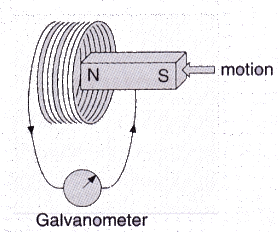Solution : (i) Electromagnetic induction.
(ii) The galvanometer gives a reading to the left.
(iii) Large deflection to right occurs more quickly.

Question 39: If you hold a coil of wire next to a magnet, no current will flow in the coil. What else is needed to induce a current ?

Solution : Relative motion between the coil and the magnet.

Question 40: The wire in Figure below is being moved downwards through the magnetic field so as to produce induced current.
What would be the effect of :
(a) moving the wire at a higher speed ?
(b) moving the wire upwards rather than downwards ?
(c) using a stronger magnet ?
(d) holding the wire still in the magnetic field ?
(e) moving the wire parallel to the magnetic field lines ?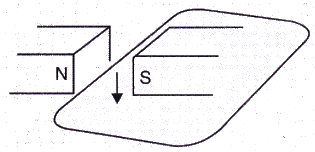Solution : (a) Current increased.
(b) Current reversed.
(c) Current increased.
(d) Zero current.
(e) Zero current.

Question 41: Two coils A and B of insulated wire are kept close to each other. Coil A is connected to a galvanometer while coil B is connected to a battery through a key. What would happen if :
(i) a current is passed through coil B by plugging the key ?
(ii) the current is stopped by removing the plug from the key ?

Solution : (i) Galvanometer pointer moves to one side showing that a momentary current is induced in the coil A.
(ii) Galvanometer pointer moves to the other side showing that the direction of momentarily induced current has been reversed.
The phenomenon taking place here is electromagnetic induction. When the current is passed through coil B or is stopped, the magnetic field linked with coil A changes due to which an induced current is produced in the coil.

Question 42: A portable radio has a built-in transformer so that it can. work from the mains instead of batteries. Is this a step-up or step down transformer ?

Solution : Step-down transformer (which reduces the voltage).

Page No:113

Question 1: What name is given to the device which automatically cuts off the electricity supply during short-circuiting in household wiring ?

Solution : Electric fuse.

Question 2: What is the usual capacity of an electric fuse used (z) in the lighting circuit, and (ii) in the power circuit, of a small house ?

Solution : (i) 5A
(ii) 15A.

Question 3: Give the symbol of an electric fuse used in circuit diagrams.

Solution :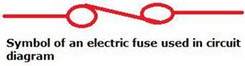Question 4: State whether the following statements are true or false :
(a) A wire with a green insulation is usually the live wire.
(b) A miniature circuit breaker (MCB) works on the heating effect of current.

Solution :
(a) False.
(b) False.

Question 5: Along with live wire and neutral wire, a third wire is also used in domestic electric wiring. What name is given to this third wire ?

Solution : Earth wire

Question 6: List the colours of the three wires in the cable connected to the plug of an electric iron.

Solution : Red wire – Live wire.
Black wire – Neutral wire.
Green wire – Earth wire.

Question 7: What is the electric potential of the neutral wire in a mains supply cable ?

Solution : Zero volt.

Question 8: If fuses of 250 mA, 500 mA, 1 A, 5 A and 10 A were available, which one would be the most suitable for protecting an amplifier rated at 240 V, 180 W ?

Solution :
P = VI
I = P/V = 180/240 = 0.75A
The fuse wire should be such that it is able to withstand only a little more current than 0.75A. So the fuse of 1A is the most suitable.

Question 9: When does an electric short circuit occur ?

Solution : Short circuit occurs when live wire and neutral wire come in contact with each other.

Question 10: In which wire in an A.C. housing circuit is the switch introduced to operate the lights ?

Solution : Live wire.

Question 11: In household circuits, is a fuse wire connected in series or in parallel ?

Solution : In series.

Question 12: Usually three insulated wires of different colours are used in an electrical appliance. Name the three colours.

Solution : Red, black and green.

Question 13: What is the usual colour of the insulation of: (a) live wire, (b) neutral wire, and (c) earth wire ?

Solution : (a)red
(b)black
(c)green

Question 14: What is the main purpose of earthing an electrical appliance ?

Solution : To avoid the risk of electric shocks.

Question 15: Give two reasons why different electrical appliances in a domestic circuit are connected in parallel.

Solution : (i) In case of parallel connection, if one of the appliances is switched off, other appliances keep on operating.
(ii) In case of parallel connection, all the appliances are operated on same voltage i.e., the mains supply voltage.

Question 16: How should the electric lamps in a building be connected so that the switching on or off in a room has no effect on other lamps in the same building ?

Solution : Parallel connection.

Question 17: Fill in the following blanks with suitable words :
(a) A fuse should always be placed in the……. wire of a mains circuit.
(b) The earth wire should be connected to the……. of an appliance.

Solution :(a) live (b) body.

Question 18: (a) Of what substance is the fuse wire made ? Why ?
(b) Explain why, a copper wire cannot be used as a fuse wire.

Solution : (a) The fuse wire is made of tin-plated copper wire because of its low melting point.
(b) Pure copper wire cannot be used as a fuse wire because it has a high melting point due to which it will not melt easily when a short circuit takes place.

Question 19: What type of electric fuse is used in electrical appliances like car stereos ? Explain with the help of a labelled diagram.

Solution : Cartridge fuse.
It consists of a glass tube T having a thin fuse wire sealed inside it. The glass tube has two metal caps at its two ends. The two ends of the fuse wire are connected to these metal caps. The metal caps are for connecting the fuse in the circuit in a suitably made bracket.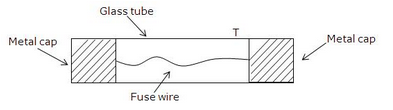Question 20: Distinguish between the terms ‘overloading’ and ‘short-circuiting ‘ as used in domestic circuits.

Solution : Live wire coming in contact with the neutral wire is known as short circuit.
When too many electrical appliances of high power rating are switched on at the same time or are connected to a single socket, they draw extremely large current. This is known as overloading.

Question 21: (a) When does a fuse cut off current ? How does it do it ?
(b) What is the maximum number of 60 W bulbs that can be run from the mains supply of 220 volts if you do not want to overload a 5 A fuse ?

Solution : (a) A fuse cuts off current when the current exceeds a safe value (due to short circuiting or overloading). When the current becomes large, it heats the fuse wire too much. Since the melting point of fuse wire is low, it melts and breaks the circuit. Thus, current in the circuit is cut off.
(b) Let the maximum number of bulbs be y.
Power of y bulbs, P=60y
V=220V, I=5A
We know that
P = VI
60y = 220 x 5
60y = 1100
y=18.33
So, number of bulbs required are 18.

Question 22: Explain the importance of using in a household electric circuit (i) fuse, and (ii) earthing wire.

Solution : (i) A fuse is one of most important protection devices, which is used for avoiding the damages happening due to over load or short circuit.
(ii) An earthing wire is used to save us from the risk of electric shock in case the live wire touches the metal case of the electric appliance.

Question 23: (a) An electric iron is rated at 230 V, 750 W. Calculate (i) the maximum current, and (ii) the number of units
of electricity it would use in 30 minutes.
(b) Which of the following fuse ratings would be suitable for this electric iron ?
1 A, 3 A, 5 A, 13 A

Solution : (a) V = 230V, P = 750 W, t = 30/60 = 0.5hr
(i) Let max current be I
We have P = VI
750 = 230xI
I = 3.26 A
(ii) Electric energy consumed, E = Pxt = 0.75kW x 0.5h = 0.375 kWh
No. of units used in 30 min = 0.375
(b) 5 A fuse rating will be suitable for this electric iron as the maximum current for this iron is 3.26 A.

Question 24: What is the function of an earth wire ? Why is it necessary to earth the metallic bodies of electrical appliances ?

Solution : When the live wire of a faulty appliance comes in direct contact with its metallic case, which has been earthed, the large current passes directly to the earth without passing through the user’s body. Thus, it is necessary to earth the metallic bodies of electrical appliances so as to avoid fatal electric shocks.

Question 25: (a) What current is taken by a 3 kW electric geyser working on 240 V mains ?
(b) What size fuse should be used in the geyser circuit ?

Solution : (a) P = 3kW = 3000W
V = 240V
P = V x I
I = P/V
= 3000/240 = 12.5 A.
(b) A 13A fuse should be used in the geyser circuit.

Question 26: (a) Why are fuses fitted in the fuse box of a domestic electricity supply ?
(b) What device could be used in place of the fuses ?

Solution : (a) Fuses are fitted in the fuse box of a domestic electricity supply to protect the whole wiring of the house when excessive current flows in the circuit.
(b) MCB.

Question 27: (a) Draw a labelled diagram to show the domestic electric wiring from an electric pole to a room. Give the
wiring for a bulb and a three-pin socket only.
(b) State two hazards associated with the use of electricity.
(c) State the important precautions which should be observed in the use of electricity.
(d) What will you do if you see a person coming in contact with a live wire ?
(e) Explain why, electric switches should not be operated with wet hands.

Solution :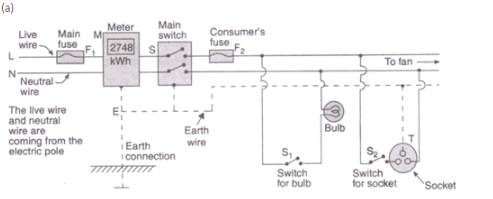(b) Two hazards associated with the use of electricity are:
i. If a person happens to touch a live electric wire, he gets a severe electric shock.
ii. Short-circuiting due to damanged wiring or overloading of the circuit can cause electrical fire in a building.
(c) Important precautions which should be observed in the use of electricity are:
(i) Use of good quality wires
(ii) Use of fuse and proper earthing.
(iii) Use of appliances in dry condition only.
(d) If a person comes in contact with a live wire, we will switch off the main switch immediately so as to cut off the electricity supply..
(e) Electric switches should not be operated with wet hands because water is a good conductor of electricity, so the user may get electric shock.

Question 38: An air-conditioner of 3.2 kW power rating is connected to a domestic electric circuit having a current rating of 10 A. The voltage of power supply is 220 V. What will happen when this air-conditioner is switched on ?

Solution : Given: P=3.2kW=3200W, Fuse current rating=10 A, V=220 V
We have
P=VI
3200 = 220 x I
I=14.54 A
As the required current for the air-conditioner is 14.54A and the rated current of the fuse is 10A, so the fuse will blow cutting off the power supply.

Question 39: Three appliances are connected in parallel to the same source which provides a voltage of 220 V. A fuse connected to the source will blow if the current from the source exceeds 10 A. If the three appliances are rated at 60 W, 500 W and 1200 W at 220 V, will the fuse blow ?

Solution : P1 = 60 W, P2 = 1200W, P3 = 500W
Fuse rating = 10A
V = 220V
We have, P=VI
Total power = 60+1200+500 = 1760W
Therefore, 1760 = 220xI
I = 8A
The required current is 8A and fuse rating is 10A. So, all the appliances will work normally and the fuse will not blow.

Question 40: A vacuum cleaner draws a current of 2 A from the mains supply.
(a) What is the appropriate value of the fuse to be fitted in its circuit ?
(b) What will happen if a 13 A fuse is fitted in its circuit ?

Solution : (a) The appropriate value of the fuse to be fitted in the circuit is 2.5A or 3A.
(b) If a 13A fuse is fitted in the circuit, it will not protect the vacuum cleaner against the very high current flowing through it. This may damage the appliance.

Question 41: Which of the following circuits will still be dangerous even if the fuse blows off and electric iron stops working during a short circuit ?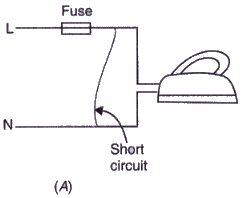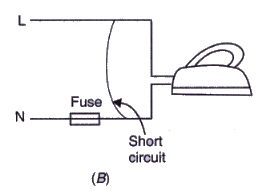Solution : Circuit A is not dangerous after fuse blows because fuse is fitted in the live wire; but circuit B is dangerous even if fuse blows because the fuse is in the neutral wire.

Page No:116

Question 42: An electric kettle rated as 1200 W at 220 V and a toaster rated at 1000 W at 220 V are both connected in parallel to a source of 220 V. If the fuse connected to the source blows when the current exceeds 9.0 A, can both appliances be used at the same time ? Illustrate your answer with calculations.

Solution : P1 = 1200W, P2 = 1000W
V = 220V
Fuse rating = 9A
We know, P = VI
Total current required,
I = P/V
= (P1+P2)/V
= (1200+1000)/220
= 10A
If both the appliances are switched on together, the fuse will get burnt. So, both the appliance cannot be used at same time.

Question 43: What is the main difference in the wiring of an electric bulb and a socket for using an electric iron in a domestic electric circuit ? What is the reason for this difference ?

Solution : No earth connection is required for the bulb connection as it does not draw heavy current and we hardly touch it. A socket for using an electric iron has an earth connection because electric iron has a metallic body and draws a large current.

Question 44: (a) Explain why, it is more dangerous to touch the live wire of a mains supply rather than the neutral wire.
(b) Why is it safe for birds to sit on naked power lines fixed atop tall electric poles ?

Solution : (a) It is more dangerous to touch the live wire rather than the neutral wire because live wire has a high potential of 220V, where as neutral wire has zero potential.
(b) Bird’s body is not connected to the earth, so no current flows through its body into the earth. So, it is safe for birds to sit on naked power lines fixed atop tall electric poles.

Question 45: A domestic lighting circuit has a fuse of 5 A. If the mains supply is at 230 V, calculate the maximum number of 36 W tube-lights that can be safely used in this circuit.

Solution : Let the maximum number of tube-lights be y.
Power of y tube-lights, P = 36y
V = 230V, I = 5A
We know that
P = VI
36y = 230 x 5
36y = 1500
y = 31.94
So, number of tube-lights required are 31.

Offer running on EduRev: Apply code STAYHOME200 to get INR 200 off on our premium plan EduRev Infinity!

61 docs

,

,

,

,

,

,

,

,

,

,

,

,

,

,

,

,

,

,

,

,

,

,

,

,

;